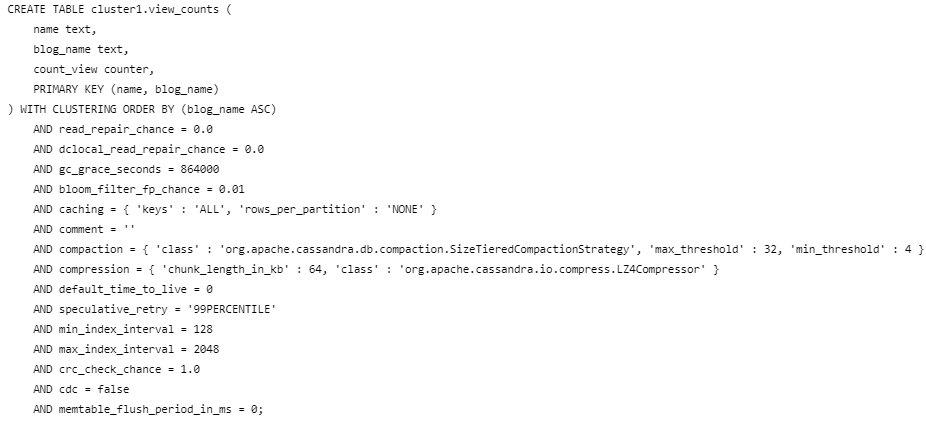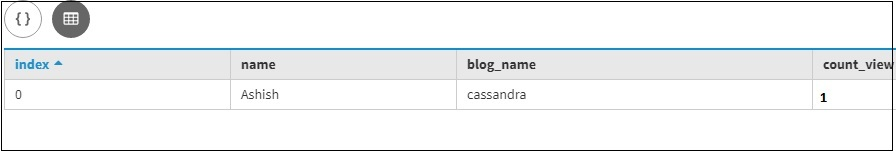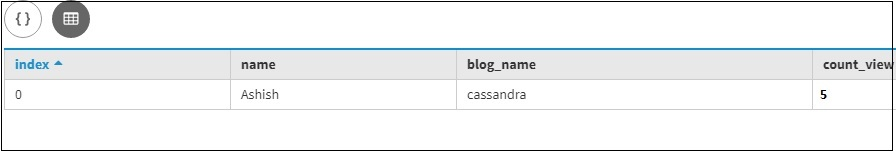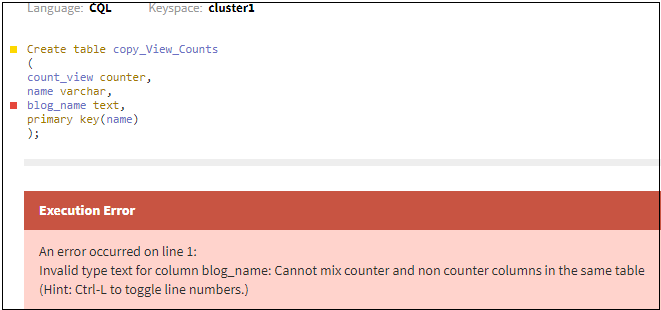# Counter Type in Cassandra

• Last Updated : 27 Dec, 2019

In this article, we will discuss how to create counter type column in Cassandra and what are the restriction while using the counter column as a data type into the table and we will see how we can update the Counter Column by using an Update Query. let’s discuss one by one.

The counter is a special column used to store a number that this changed increments. For example, you might use a counter column to count the number of times a page is viewed. So, we can define a counter in a dedicated table only and use that counter datatype.

Restriction on the counter column:

• Counter column cannot index, delete or re-add a counter column.
• All non-counter columns in the table must be defined as a part of the primary key.
• To load data in a counter column or to increase or decrease the value of the counter, use the update command.

Now, we are going to create table with a Counter column. let’s have a look.

```Create table View_Counts
(
count_view counter,
name varchar,
blog_name text,
primary key(name, blog_name)
); ```

Let’s see the table schema.

`describe table View_Counts; `

Output:Now, we are going to insert value for the counter column with the help of the Update clause.
Let’s have a look.

```update View_counts set count_view = count_view+1
where name = 'Ashish'and blog_name =  'cassandra';  ```

Let’s see the result.

```select *
from View_Counts; ```

Output:Now, we are going to update the value for the counter column with the help of the Update clause.
Let’s have a look.

```update View_counts set count_view = count_view + 4
where name = 'Ashish'and blog_name =  'cassandra'; ```

Let’s see the result.

```select *
from View_Counts; ```

Output:We can also decrement the value for the counter column with the help of the Update clause. let’s have a look.

```update View_counts set count_view = count_view - 4
where name = 'Ashish'and blog_name =  'cassandra'; ```

Let’s see the result.

```select *
from View_Counts; ```

Output:Cassandra rejects using time star or using TTL in the command to update a counter column. So, let’s create a View_Counts table. So, we created count_view and this is a counter value of type counter. This is very important and we have a name and blog_name and we can see that the primary key is to have a name and blog_name.

If we will try to create a similar table, let’s say – copy_View_Counts and the primary key will be the only name, we’ll get an error. Because cannot mix counter and non-counter columns in the same table if they are not primary key. let’s have a look.

Let’s understand with an example.

```Create table copy_View_Counts
(
count_view counter,
name varchar,
blog_name text,
primary key(name)
); ```

Here, All non-counter columns must be part of the primary key. but, the only name is the only part of primary column that’s why it is giving an error. let’s have a look.

Let’s see the result.

```select *
from View_Counts; ```

Output:My Personal Notes arrow_drop_up